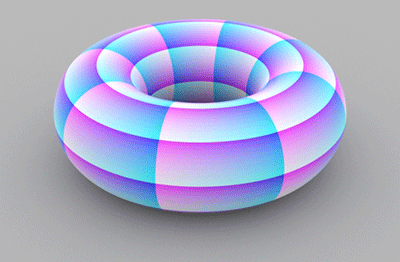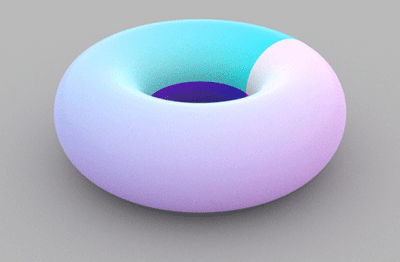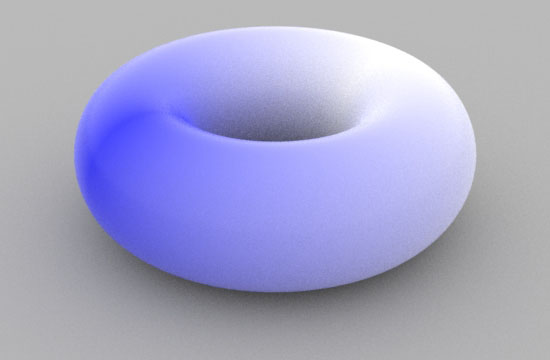### OSLTo UV or not to UV

#### Introduction

OSL shaders written for use with rendering systems such a VRay can directly refer to 'uv' texture coordinates when generating a surface pattern. However, using 'u' and 'v' with RenderMan will not work as expected. For example, figure 1 shows the effect of using a shader called "use_uv" (listing 1) on a nurbs torus with 8 x 8 "divisions".Figure 1 - using 'uv' coordinates

Listing 1 - use_uv.osl

 ```shader use_uv(output color resultRGB = 0) { resultRGB = color(u, v, 1); }```

RenderMan uses it's own unique texture coordinate system, designated 'st', and as such OSL shaders that generate 2D texture patterns must reference 's' and 't' as primvars. Some basic information about primvars can be found in the tutorial "RIB: Introduction to Primvars". An OSL shader that uses 'st' coordinates for a surface pattern (listing 2) produces the correct effect - figure 2 (listing 2).Figure 2 - using 'st' coordinates

Listing 2 - use_st.osl

 ```shader use_st( float s = 0 [[int lockgeom = 0]], float t = 0 [[int lockgeom = 0]], output color resultRGB = 0) { resultRGB = color(s, t, 1); }```

Note the use of the metadata tag.
`[[int lockgeom = 0]]`

This tells the renderer the value of a shader (input) parameter will be provided by data associated with the geometry. The data is called a "primvar".

#### Avoiding 's' & 't' Seams

Instead of using the 'st' texture coordinates directly their values can remapped so that the "seams" where 's' and 't' wrap from 0.0 to 1.0 can be avoided. For example, listing 3 and figure 3.

Listing 3 - use_st.osl (remapped)

 ```shader use_st( float s = 0 [[int lockgeom = 0]], float t = 0 [[int lockgeom = 0]], output color resultRGB = 0) { float ss = abs(s - 0.5) * 2; float tt = abs(s - 0.5) * 2; resultRGB = color(ss, tt, 1); } ```Figure 3